Author: Oscar Cronquist Article last updated on November 06, 2018

Question: How do I sort a range alphabetically using excel array formula?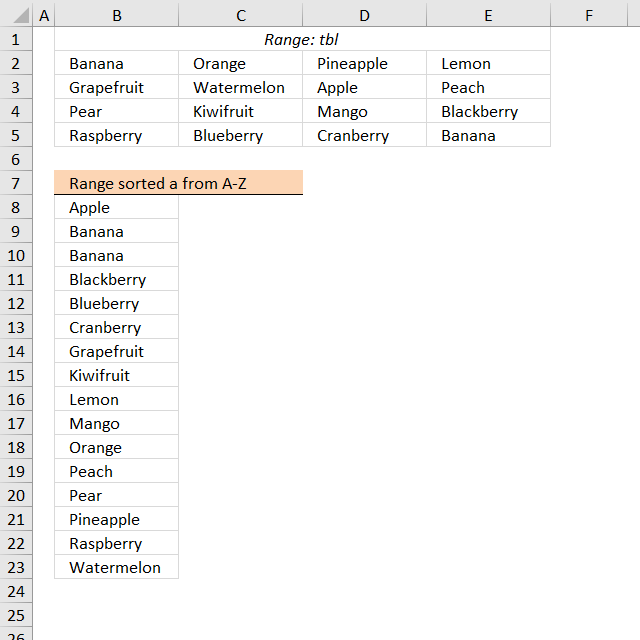Cell range \$B\$2:\$E\$5 contains text values in random order, the formula in cell B8 extracts values sorted from A to Z.

Array formula in B8:

=INDEX(\$B\$2:\$E\$5, MIN(IF(SMALL(COUNTIF(\$B\$2:\$E\$5, "<"&\$B\$2:\$E\$5), ROWS(\$B\$8:B8))=COUNTIF(\$B\$2:\$E\$5, "<"&\$B\$2:\$E\$5), ROW(\$B\$2:\$E\$5)-MIN(ROW(\$B\$2:\$E\$5))+1)), MATCH(SMALL(COUNTIF(\$B\$2:\$E\$5, "<"&\$B\$2:\$E\$5), ROWS(\$B\$8:B8)), COUNTIF(\$B\$2:\$E\$5, "<"&INDEX(\$B\$2:\$E\$5, MIN(IF(SMALL(COUNTIF(\$B\$2:\$E\$5, "<"&\$B\$2:\$E\$5), ROWS(\$B\$8:B8))=COUNTIF(\$B\$2:\$E\$5, "<"&\$B\$2:\$E\$5), ROW(\$B\$2:\$E\$5)-MIN(ROW(\$B\$2:\$E\$5))+1)), , 1)), 0))

Filter unique distinct values sorted from A to Z with no blanks from a multi-column and multi-row cell range:

Recommended articles

Extract unique distinct values A to Z from a range and ignore blanks
This article demonstrates formulas that extract sorted unique distinct values from a cell range containing also blanks. Unique distinct values […]

#### How to enter an array formula

1. Double press with left mouse button on cell B8
2. Copy an paste above formula
3. Press and hold CTRL + SHIFT simultaneously
4. Press Enter once

Learn how to filter unique distinct values from a multi-column and multi-row cell range:

Recommended articles

Extract unique distinct values from a multi-column cell range
Question: I have cell values spanning over several columns and I want to create a unique list from that range. […]

#### How to implement array formula to your workbook

If your list starts at, for example, F2. Change \$B\$8:B8  in the above formula to F2:\$F\$2.

The following article demonstrates how to filter a unique distinct list sorted from A to Z, from a multi-column and multi-row cell range:

Recommended articles

Extract a unique distinct list sorted from A-Z from range
The image above shows an array formula in cell B8 that extracts unique distinct values sorted alphabetically from cell range […]

#### How to copy array formula

Copy cell B8 and paste to cells below.

How to filter duplicate values from a multi-column and multi-row cell range:

Recommended articles

Extract duplicates from a multi-column cell range
This article describes two formulas that extract duplicates from a multi-column cell range, the first one is built for Excel […]

### Explaining formula in cell B8

#### Step 1 - Rank values based on their relative position if they were sorted

The COUNTIF function counts cells based on a condition, however, in this case, I am using a cell range instead of a single cell in the second argument.

Also, the ampersand & concatenates each value with a less than sign. This makes the COUNTIF function compare each value against the others, the number returned represents the corresponding values position if the list were sorted.

COUNTIF(\$B\$2:\$E\$5,"<"&\$B\$2:\$E\$5)

returns {1, 10, 13, 8; 6, 15, 0, 11; 12, 7, 9, 3; 14, 4, 5, 1}.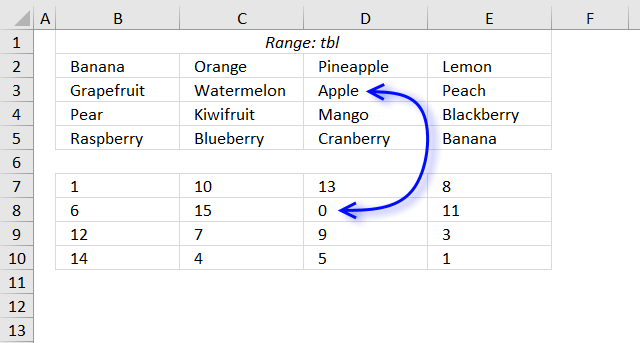The image above shows the array in cell range B7:E10, "Apple" is 0 (zero) which is the first position if the list were sorted.

#### Step 2 - Extract the k-th smallest value in array

The SMALL function lets you get the k-th smallest value from a cell range or array.

SMALL(array, k)

SMALL(COUNTIF(\$B\$2:\$E\$5, "<"&\$B\$2:\$E\$5), ROWS(\$B\$8:B8))

The ROWS function allows you to keep track of the number of cells, the cell reference is expanding as we copy the formula and paste to cells below.

SMALL({1, 10, 13, 8; 6, 15, 0, 11; 12, 7, 9, 3; 14, 4, 5, 1}, ROWS(\$B\$8:B8))

becomes

SMALL({1, 10, 13, 8; 6, 15, 0, 11; 12, 7, 9, 3; 14, 4, 5, 1}, 1) and returns 0 (zero).

#### Step 3 - Compare value to array

The logical expression in the following IF function determines if the value of the position corresponding to the k-th smallest value will be a row number or FALSE.

IF(SMALL(COUNTIF(\$B\$2:\$E\$5, "<"&\$B\$2:\$E\$5), ROWS(\$B\$8:B8))=COUNTIF(\$B\$2:\$E\$5, "<"&\$B\$2:\$E\$5), ROW(\$B\$2:\$E\$5)-MIN(ROW(\$B\$2:\$E\$5))+1)

becomes

IF(0=COUNTIF(\$B\$2:\$E\$5, "<"&\$B\$2:\$E\$5), ROW(\$B\$2:\$E\$5)-MIN(ROW(\$B\$2:\$E\$5))+1)

becomes

IF(0={1, 10, 13, 8; 6, 15, 0, 11; 12, 7, 9, 3; 14, 4, 5, 1}, ROW(\$B\$2:\$E\$5)-MIN(ROW(\$B\$2:\$E\$5))+1)

becomes

IF({FALSE, FALSE, FALSE, FALSE;FALSE, FALSE, TRUE, FALSE;FALSE, FALSE, FALSE, FALSE;FALSE, FALSE, FALSE, FALSE}, ROW(\$B\$2:\$E\$5)-MIN(ROW(\$B\$2:\$E\$5))+1)

becomes

IF({FALSE, FALSE, FALSE, FALSE;FALSE, FALSE, TRUE, FALSE;FALSE, FALSE, FALSE, FALSE;FALSE, FALSE, FALSE, FALSE}, {1;2;3;4})

and returns {FALSE, FALSE, FALSE, FALSE;FALSE, FALSE, 2, FALSE;FALSE, FALSE, FALSE, FALSE;FALSE, FALSE, FALSE, FALSE}.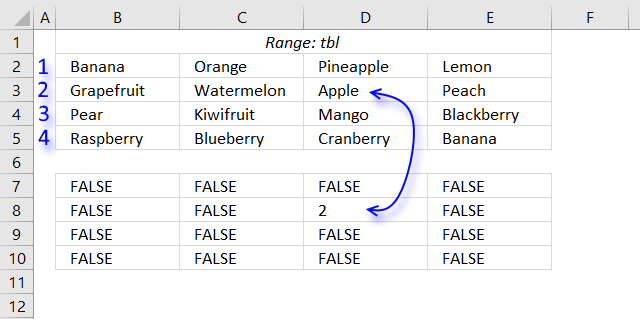#### Step 4 - Extract the smallest number from array

The MIN function ignores boolean values TRUE and FALSE.

MIN(IF(SMALL(COUNTIF(\$B\$2:\$E\$5, "<"&\$B\$2:\$E\$5), ROWS(\$B\$8:B8))=COUNTIF(\$B\$2:\$E\$5, "<"&\$B\$2:\$E\$5), ROW(\$B\$2:\$E\$5)-MIN(ROW(\$B\$2:\$E\$5))+1))

becomes

MIN({FALSE, FALSE, FALSE, FALSE;FALSE, FALSE, 2, FALSE;FALSE, FALSE, FALSE, FALSE;FALSE, FALSE, FALSE, FALSE})

and returns 2. We now know that the first value to be extracted is located on row 2 relative to cell range \$B\$2:\$E\$5.

#### Step 5 - Find column

In this case, the MATCH function returns the relative position horizontally of the value we are looking for.

MATCH(SMALL(COUNTIF(\$B\$2:\$E\$5,"<"&\$B\$2:\$E\$5),ROWS(\$B\$8:B8)),COUNTIF(\$B\$2:\$E\$5,"<"&INDEX(\$B\$2:\$E\$5,MIN(IF(SMALL(COUNTIF(\$B\$2:\$E\$5,"<"&\$B\$2:\$E\$5),ROWS(\$B\$8:B8))=COUNTIF(\$B\$2:\$E\$5,"<"&\$B\$2:\$E\$5),ROW(\$B\$2:\$E\$5)-MIN(ROW(\$B\$2:\$E\$5))+1)),,1)),0)

becomes

MATCH(SMALL({1, 10, 13, 8; 6, 15, 0, 11; 12, 7, 9, 3; 14, 4, 5, 1},ROWS(\$B\$8:B8)),COUNTIF(\$B\$2:\$E\$5,"<"&INDEX(\$B\$2:\$E\$5,MIN(IF(SMALL(COUNTIF(\$B\$2:\$E\$5,"<"&\$B\$2:\$E\$5),ROWS(\$B\$8:B8))=COUNTIF(\$B\$2:\$E\$5,"<"&\$B\$2:\$E\$5),ROW(\$B\$2:\$E\$5)-MIN(ROW(\$B\$2:\$E\$5))+1)),,1)),0)

becomes

MATCH(SMALL({1, 10, 13, 8; 6, 15, 0, 11; 12, 7, 9, 3; 14, 4, 5, 1},1),COUNTIF(\$B\$2:\$E\$5,"<"&INDEX(\$B\$2:\$E\$5,MIN(IF(SMALL(COUNTIF(\$B\$2:\$E\$5,"<"&\$B\$2:\$E\$5),ROWS(\$B\$8:B8))=COUNTIF(\$B\$2:\$E\$5,"<"&\$B\$2:\$E\$5),ROW(\$B\$2:\$E\$5)-MIN(ROW(\$B\$2:\$E\$5))+1)),,1)),0)

becomes

MATCH(0,COUNTIF(\$B\$2:\$E\$5,"<"&INDEX(\$B\$2:\$E\$5,MIN(IF(SMALL(COUNTIF(\$B\$2:\$E\$5,"<"&\$B\$2:\$E\$5),ROWS(\$B\$8:B8))=COUNTIF(\$B\$2:\$E\$5,"<"&\$B\$2:\$E\$5),ROW(\$B\$2:\$E\$5)-MIN(ROW(\$B\$2:\$E\$5))+1)),,1)),0)

I will be using the INDEX function to extract the values we need based on a row number.

MATCH(0,COUNTIF(\$B\$2:\$E\$5,"<"&INDEX(\$B\$2:\$E\$5,MIN(IF(SMALL(COUNTIF(\$B\$2:\$E\$5,"<"&\$B\$2:\$E\$5),ROWS(\$B\$8:B8))=COUNTIF(\$B\$2:\$E\$5,"<"&\$B\$2:\$E\$5),ROW(\$B\$2:\$E\$5)-MIN(ROW(\$B\$2:\$E\$5))+1)),,1)),0)

becomes

MATCH(0,COUNTIF(\$B\$2:\$E\$5,"<"&INDEX(\$B\$2:\$E\$5,2,,1)),0)

becomes

MATCH(0,COUNTIF(\$B\$2:\$E\$5,"<"&B3:E3),0)

becomes

MATCH(0,{6,15,0,11},0)

and returns 3. The value we are looking for is in column 3 relative to cell range \$B\$2:\$E\$5.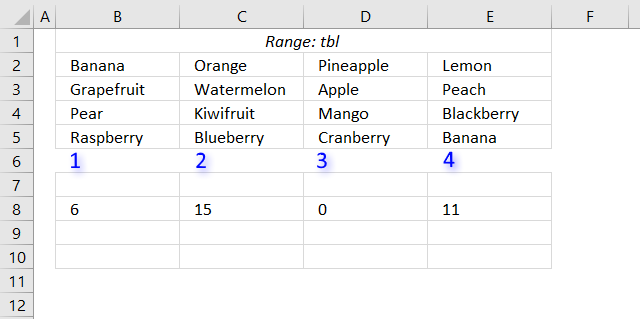#### Step 6 - Return value based on row and column

INDEX(\$B\$2:\$E\$5, MIN(IF(SMALL(COUNTIF(\$B\$2:\$E\$5, "<"&\$B\$2:\$E\$5), ROWS(\$B\$8:B8))=COUNTIF(\$B\$2:\$E\$5, "<"&\$B\$2:\$E\$5), ROW(\$B\$2:\$E\$5)-MIN(ROW(\$B\$2:\$E\$5))+1)), MATCH(SMALL(COUNTIF(\$B\$2:\$E\$5, "<"&\$B\$2:\$E\$5), ROWS(\$B\$8:B8)), COUNTIF(\$B\$2:\$E\$5, "<"&INDEX(\$B\$2:\$E\$5, MIN(IF(SMALL(COUNTIF(\$B\$2:\$E\$5, "<"&\$B\$2:\$E\$5), ROWS(\$B\$8:B8))=COUNTIF(\$B\$2:\$E\$5, "<"&\$B\$2:\$E\$5), ROW(\$B\$2:\$E\$5)-MIN(ROW(\$B\$2:\$E\$5))+1)), , 1)), 0))

becomes

INDEX(\$B\$2:\$E\$5, 2, 3)

and returns "Apple" in cell B8.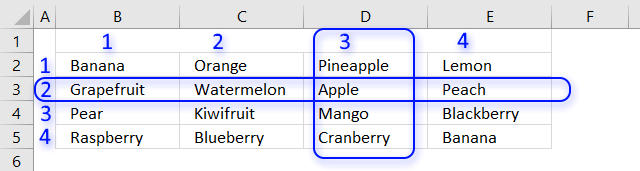### Get Excel *.xlsx file

Range sorted from A to Z.xlsx

This post shows you how to filter a multi-column and multi-row cell range based on frequency:

Recommended articles

Sort a range based on value frequency
The formula in cell B8 extracts a list sorted based on frequency. Array formula in B8: =TEXTJOIN("", TRUE, IF(MIN(IF((MAX(IF(COUNTIF(\$B\$7:B7, \$B\$2:\$E\$5)=0, […]

Recommended article

Recommended articles

A beginners guide to Excel array formulas
Array formulas allows you to do advanced calculations not possible with regular formulas.

Recommended article

Recommended articles

How to sort a data set using three different approaches, built-in tools, array formulas, and VBA
I will in this article demonstrate three different techniques to sort a data set in Excel. The first method sorts […]

This blog article is one out of five articles on the same subject.

Recommended articles

Recommended articles

Sort text cells alphabetically from two columns
Table of Contents Sort text from two columns combined (array formula) How to create an array formula How to copy […]

Recommended articles

Populate drop down list with unique distinct values sorted from A to Z
Question: How do I create a drop-down list with unique distinct alphabetically sorted values? Table of contents Sort values using […]

Recommended articles